Ex 3.3

Chapter 3 Class 12 Matrices
Serial order wise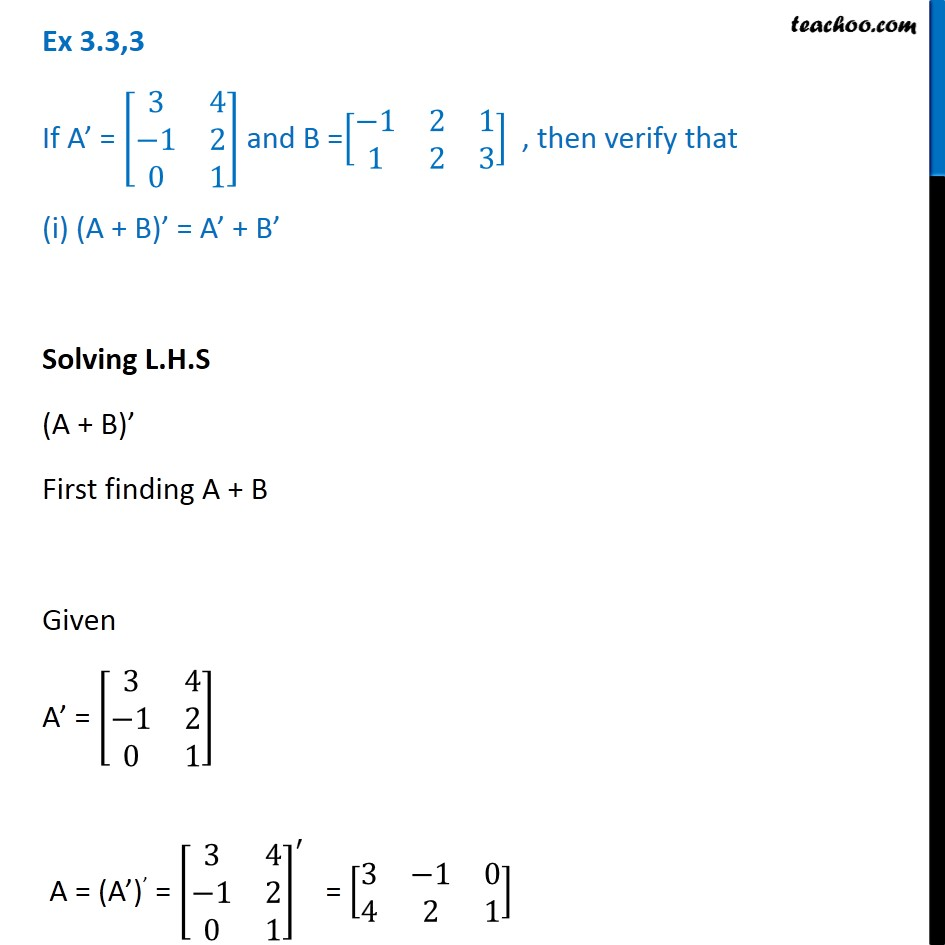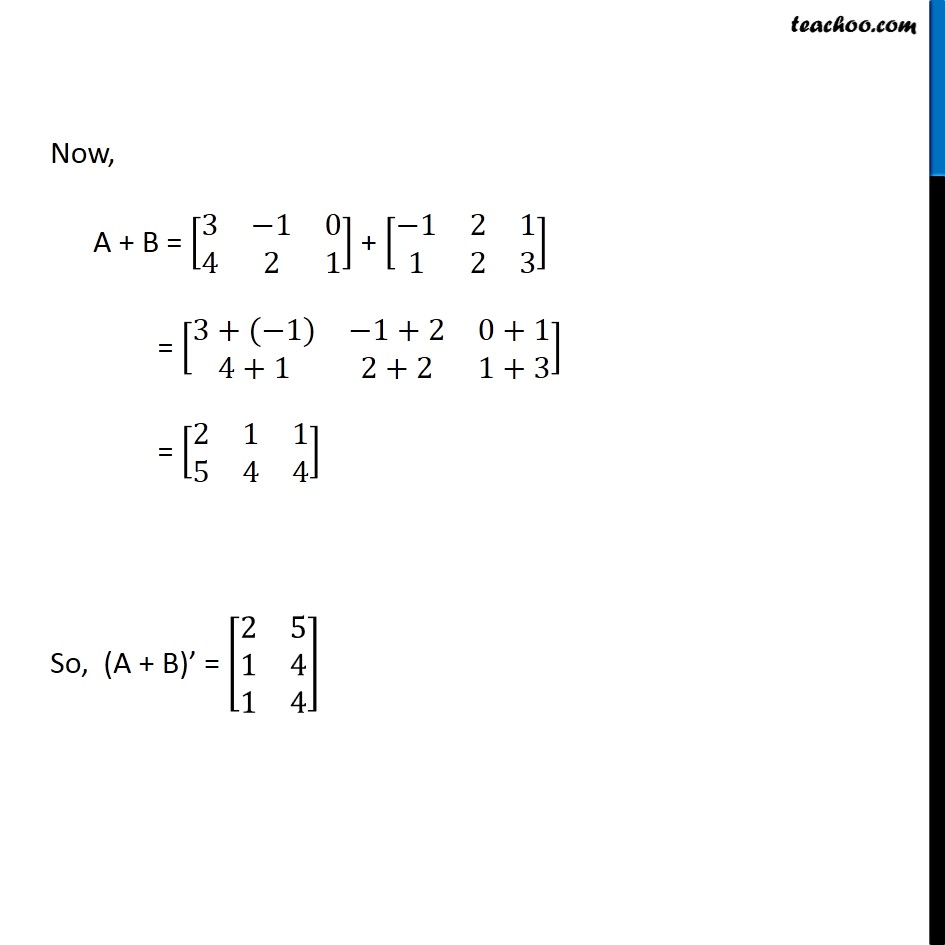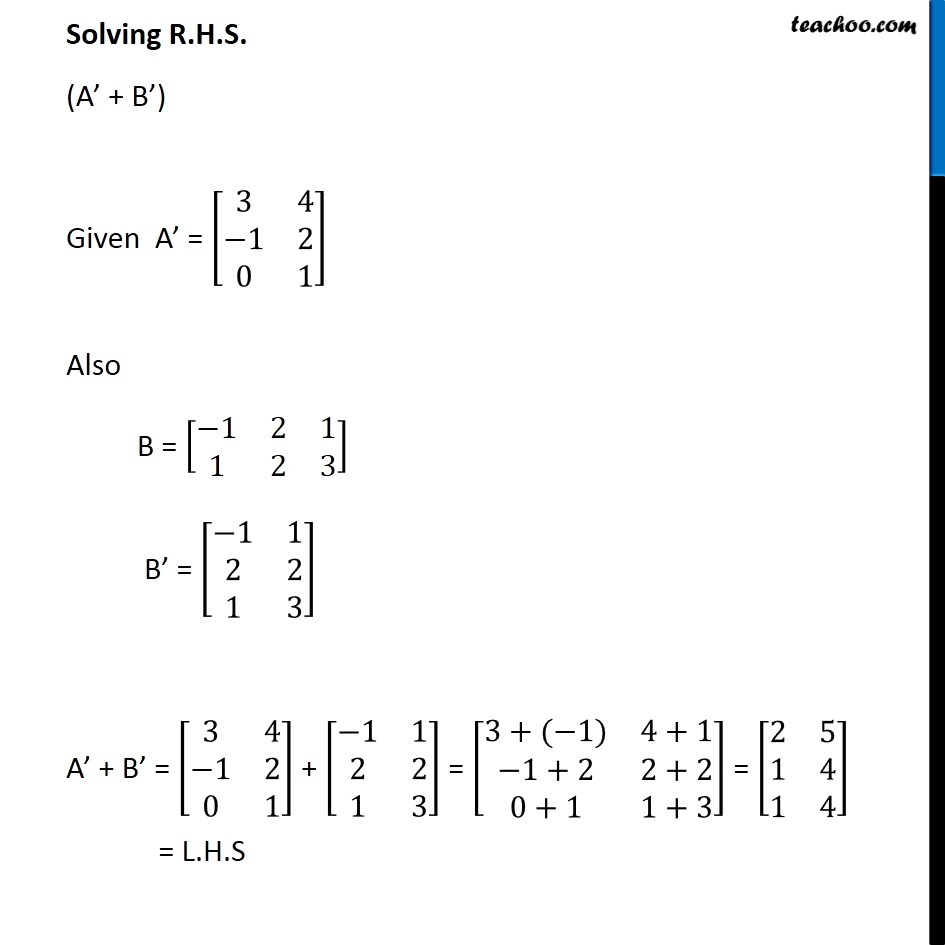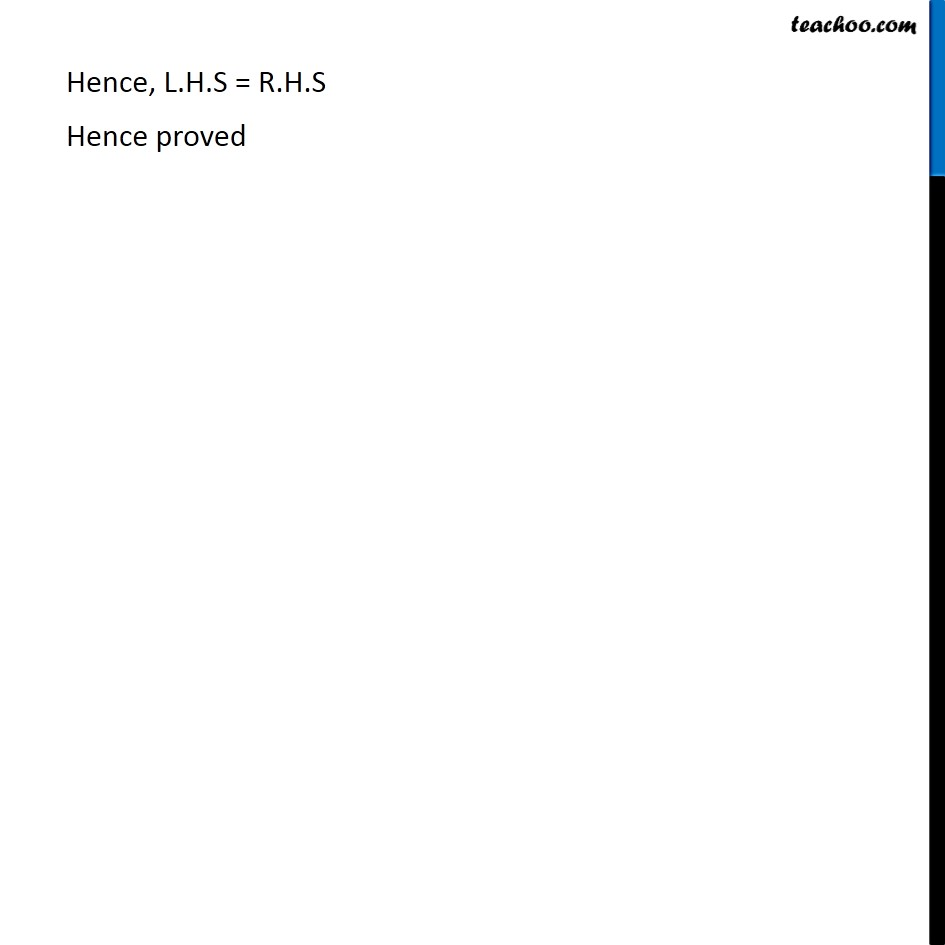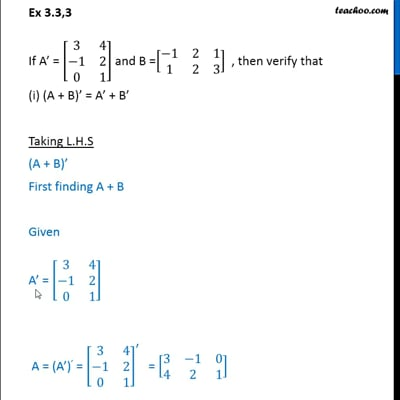This video is only available for Teachoo black users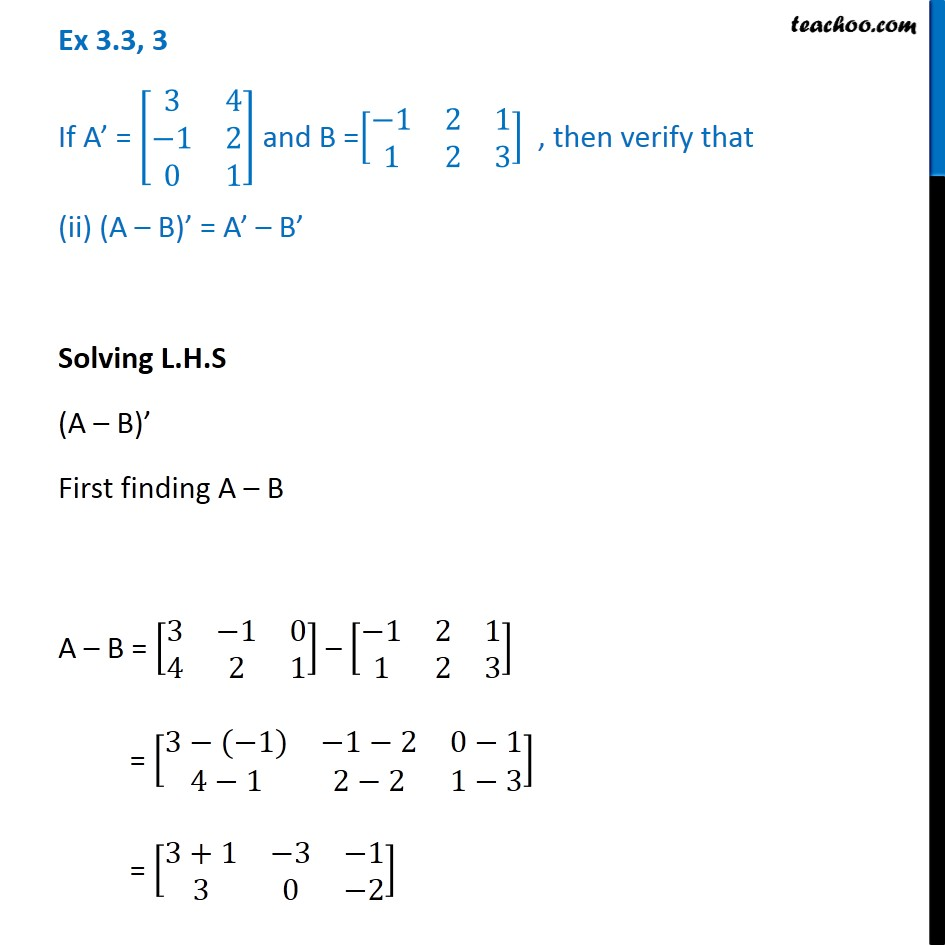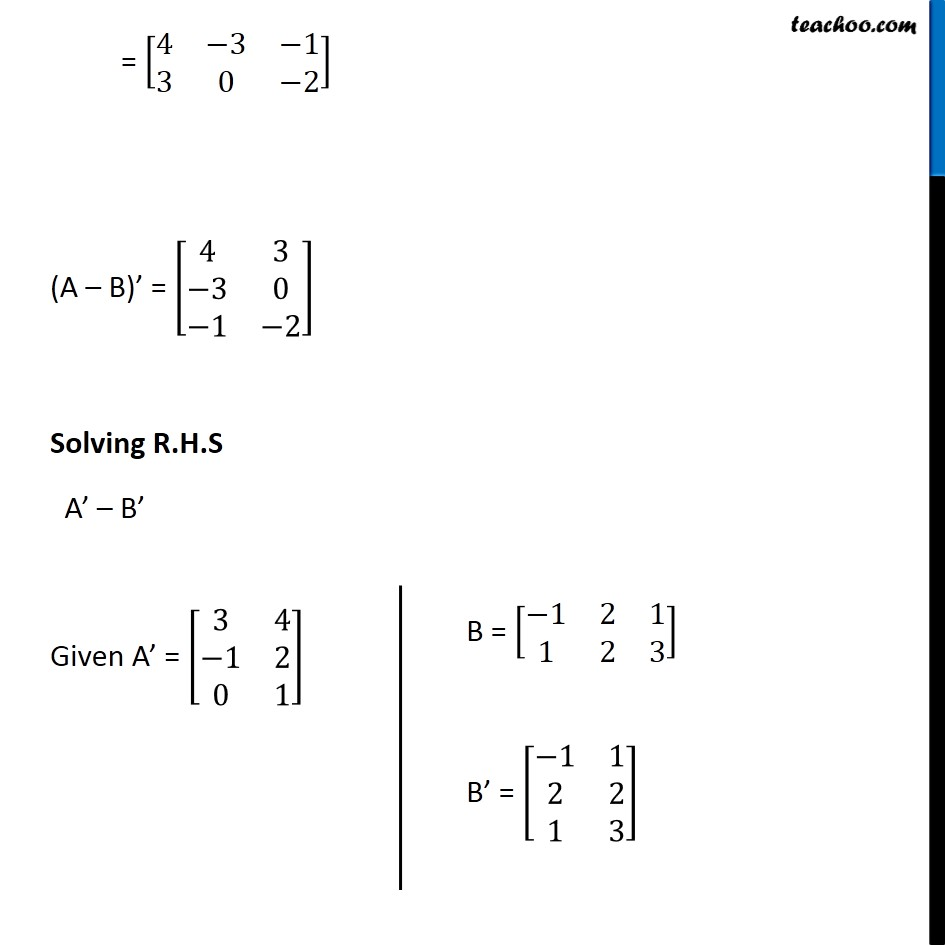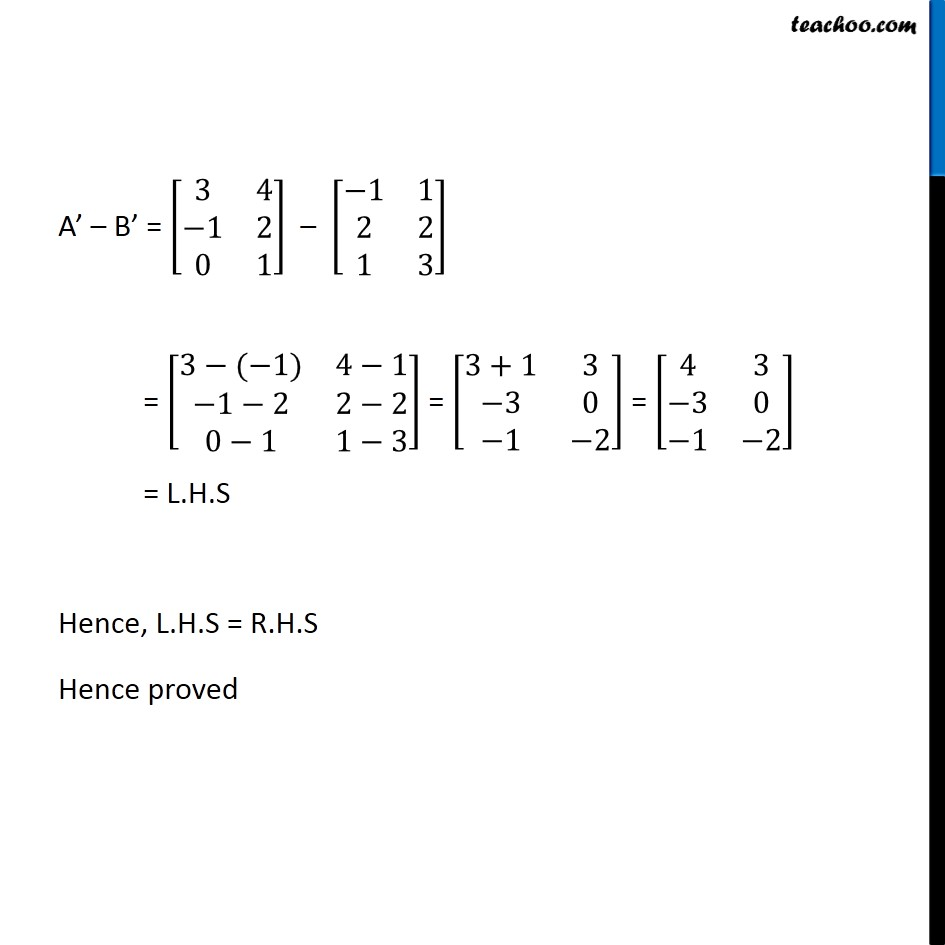Solve all your doubts with Teachoo Black (new monthly pack available now!)

### Transcript

Ex 3.3,3 If A’ = [■8(3&4@−1&2@0&1)] and B =[■8(−1&2&1@1&2&3)] , then verify that (i) (A + B)’ = A’ + B’ Solving L.H.S (A + B)’ First finding A + B Given A’ = [■8(3&4@−1&2@0&1)] A = (A’)’ = [■8(3&4@−1&2@0&1)]^′ = [■8(3&−1&0@4&2&1)] Now, A + B = [■8(3&−1&0@4&2&1)] + [■8(−1&2&1@1&2&3)] = [■8(3+(−1)&−1+2&0+1@4+1&2+2&1+3)] = [■8(2&1&1@5&4&4)] So, (A + B)’ = [■8(2&5@1&4@1&4)] Solving R.H.S. (A’ + B’) Given A’ = [■8(3&4@−1&2@0&1)] Also B = [■8(−1&2&1@1&2&3)] B’ = [■8(−1&1@2&2@1&3)] A’ + B’ = [■8(3&4@−1&2@0&1)] + [■8(−1&1@2&2@1&3)] = [■8(3+(−1)&4+1@−1+2&2+2@0+1&1+3)] = [■8(2&5@1&4@1&4)] = L.H.S Hence, L.H.S = R.H.S Hence proved Ex 3.3, 3 If A’ = [■8(3&4@−1&2@0&1)] and B =[■8(−1&2&1@1&2&3)] , then verify that (ii) (A – B)’ = A’ – B’ Solving L.H.S (A – B)’ First finding A – B A – B = [■8(3&−1&0@4&2&1)] – [■8(−1&2&1@1&2&3)] = [■8(3−(−1)&−1−2&0−1@4−1&2−2&1−3)] = [■8(3+1&−3&−1@3&0&−2)] = [■8(4&−3&−1@3&0&−2)] (A – B)’ = [■8(4&3@−3&0@−1&−2)] Solving R.H.S A’ – B’ Given A’ = [■8(3&4@−1&2@0&1)] B = [■8(−1&2&1@1&2&3)] B’ = [■8(−1&1@2&2@1&3)] A’ – B’ = [■8(3&4@−1&2@0&1)] – [■8(−1&1@2&2@1&3)] = [■8(3−(−1)&4−1@−1−2&2−2@0−1&1−3)] = [■8(3+1&3@−3&0@−1&−2)] = [■8(4&3@−3&0@−1&−2)] = L.H.S Hence, L.H.S = R.H.S Hence proved